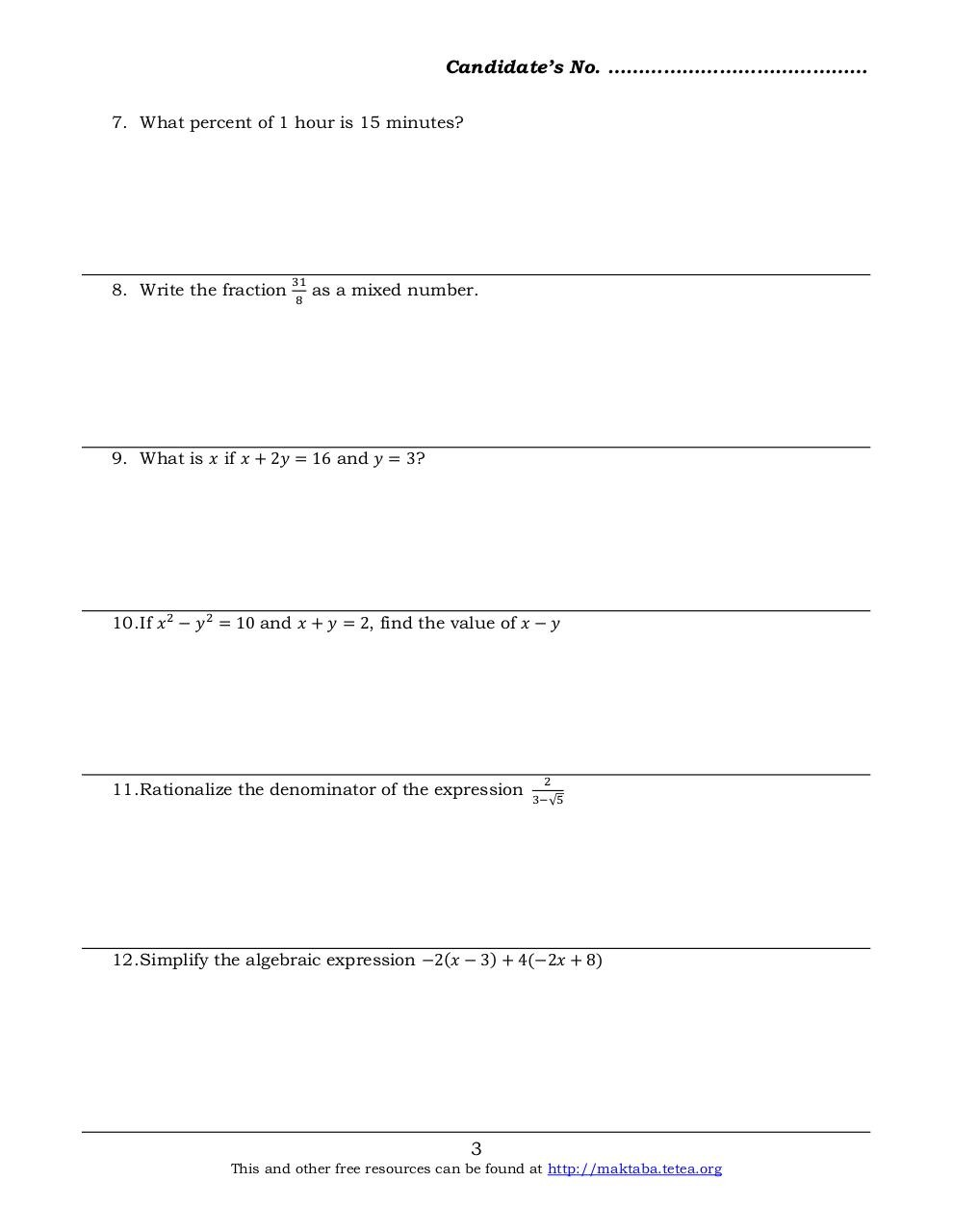# Basic Math 2013 F2.pdfPage 1 2 3 4 5 6 7 8

#### Text preview

Candidate’s No. ……………………………………
7. What percent of 1 hour is 15 minutes?

8. Write the fraction

31
8

as a mixed number.

9. What is 𝑥 if 𝑥 + 2𝑦 = 16 and 𝑦 = 3?

10. If 𝑥 2 − 𝑦 2 = 10 and 𝑥 + 𝑦 = 2, find the value of 𝑥 − 𝑦

11. Rationalize the denominator of the expression

2
3−√5

12. Simplify the algebraic expression −2(𝑥 − 3) + 4(−2𝑥 + 8)

3

This and other free resources can be found at http://maktaba.tetea.org